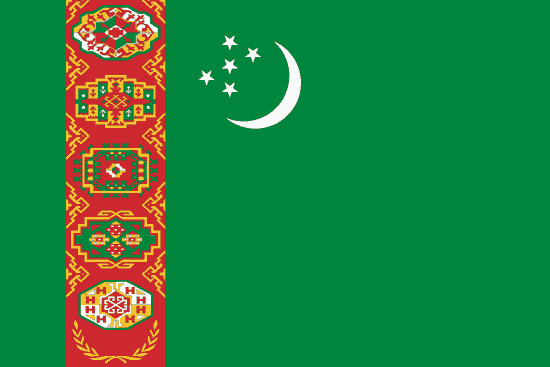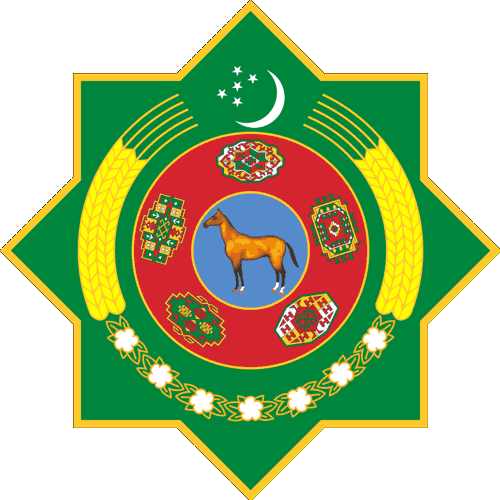1929年以前，土库曼语使用阿拉伯字母书写。1938年，西里尔字母取代了阿拉伯字母。1991年，土库曼斯坦总统尼亚佐夫颁令土库曼语改用经修改的拉丁字母书写。

 土库曼语字母 拉丁字母 相对于西里尔字母 音位 A a А а [a] B b Б б [b] C c* Ç ç Ч ч [ʧ] D d Д д [d] E e Е е [je], [e] Ä ä Ә ә [æ] F f Ф ф [ɸ] G g Г г [g~ʁ] H h Х х [h~x] I i И и [i] J j Җ җ [ʤ] Ž ž Ж ж [ʒ] K k К к [k~q] L l Л л [l] M m М м [m] N n Н н [n] Ň ň Ң ң [ŋ] O o О о [o] Ö ö Ө ө [ø] P p П п [p] Q q* R r Р р [r] S s С с [θ] Ş ş Ш ш [ʃ] T t Т т [t] U u У у [u] Ü ü Ү ү [y] V v* W w В в [β] X x* Y y Ы ы [ɯ] Ý ý Й й [j] Z z З з [ð]